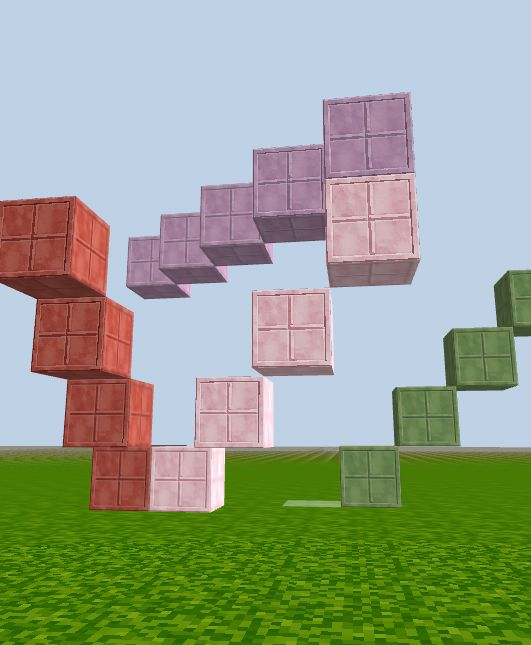### Diagonals

In addition to looping through one direction, we can also loop through two or three directions at the same time to build diagonal lines of blocks in CodeCraft world.

By using loop counter variable in place of the `x, y`, or `z` coordinates, we can build:

Face Diagonal:

``````// x-y plane (green)
for (let i = 0; i < 5; i++) {
game.setBlock(new Position(i+3, i, -20), 6);
}

// x-z plane (purple)
for (let i = 0; i < 5; i++) {
game.setBlock(new Position(i, 5, i-20), 12);
}

// y-z plane (red)
for (let i = 0; i < 5; i++) {
game.setBlock(new Position(0, i, i-20), 13);
}
``````

Space Diagonal (pink):

``````for (let i = 0; i < 5; i++) {
game.setBlock(new Position(i, i, i-20), 11);
}
``````See, you can also learn geometry!